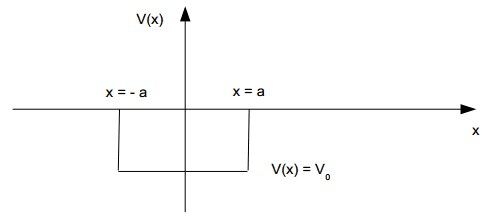# Finite potential well

feynwomann'
I've got these solutions to the Schrödinger equation (##-\frac{\hbar} {2m} \frac {d^2} {dx^2} \psi(x) + V(x)*\psi(x)=E*\psi(x)##):
x < -a: ##\psi(x)=C_1*e^(k*x)##
-a < x < a: ##\psi(x)=A*cos(q*x)+B*sin(q*x)##
x > a: ##\psi(x)=C_2*e^(-k*x)##

##q^2=\frac {2m(E+V_0)} {\hbar^2}## and ##k^2=\frac {2mE} {\hbar^2}##

In a previous exercise i showed that in order for the wavefunction to be continuous the following has to be true:
For x = -a: ##C_1*e^-(k*a)=A*cos(q*a)-B*sin(q*a)##
For x = a: ##C_2*e^-(k*a)=A*cos(q*a)+B*sin(q*a)##
and for the derived wavefunctions:
x = -a: ##k*C_1*e^-(k*a)=q*(A*sin(q*a)+B*cos(q*a))##
x = a: ##-k*C_2*e^-(k*a)=-q*(A*sin(q*a)-B*cos(q*a))##

I also know that A*B=0. So here's the question:

I'm asked to show that when A = 0, the four equations above kan be reduced to ##k=q*tan(q*a). But I'm not even sure where to start.

Last edited:

Staff Emeritus'
I've got these solutions to the Schrödinger equation (##-\frac{\hbar} {2m} \frac {d^2} {dx^2} \psi(x) + V(x)*\psi(x)=E*\psi(x)##):
x < -a: ##\psi(x)=C_1*e^(k*x)##
-a < x < a: ##\psi(x)=A*cos(q*x)+B*sin(q*x)##
x > a: ##\psi(x)=C_2*e^(-k*x)##

##q^2=\frac {2m(E+V_0)} {\hbar^2}## and ##k^2=\frac {2mE} {\hbar^2}##

In a previous exercise i showed that in order for the wavefunction to be continuous the following has to be true:
For x = -a: ##C_1*e^-(k*a)=A*cos(q*a)-B*sin(q*a)##
For x = a: ##C_2*e^-(k*a)=A*cos(q*a)+B*sin(q*a)##
and for the derived wavefunctions:
x = -a: ##k*C_1*e^-(k*a)=q*(A*sin(q*a)+B*cos(q*a))##
x = a: ##-k*C_2*e^-(k*a)=-q*(A*sin(q*a)-B*cos(q*a))##

I also know that A*B=0. So here's the question:

I'm asked to show that when A = 0, the four equations above kan be reduced to ##k=q*tan(q*a). But I'm not even sure where to start.

Sure, you do. When $A=0$, your equations become:
1. ##C_1 e^{-ka}= -B sin(qa)##
2. ##C_2 e^{-ka}=-B sin(qa)##
3. ##k C_1 e^{-ka}=q Bcos(qa))##
4. ##-kC_2*e^{-ka}=q Bcos(qa))##
Using equation 1, you know $C_1e^{-ka} = -Bsin(qa)$
So replace $C_1 e^{-ka}$ by $-B sin(qa)$ in equation 3.

It doesn't look like it results in the same formula as you are expecting, so maybe there was a mistake somewhere.

•feynwomann
feynwomann
Oh it totally makes sense now. I forgot that my teacher said there was a mistake in the exercise. It's supposed to be B = 0. I didn't think of dividing the equations (I had actually done the first step, but didn't see how I could take it further). Anyway, thanks for the help!

Last edited: